# Common Core: High School - Number and Quantity : Understanding the Multiplication Concept in Matrices as the Associative and Distributive Properties: CCSS.Math.Content.HSN-VM.C.9

## Example Questions

← Previous 1

### Example Question #1 : Understanding The Multiplication Concept In Matrices As The Associative And Distributive Properties: Ccss.Math.Content.Hsn Vm.C.9

Which of the following properties does not apply to matrices?

Commutative

Associative

Distributive

None of the answers

Commutative

Explanation:

Commutative does not apply to matrices because if we have matrices, and. It is not necessarily true that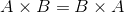, even though in some cases it's true.

### Example Question #2 : Understanding The Multiplication Concept In Matrices As The Associative And Distributive Properties: Ccss.Math.Content.Hsn Vm.C.9

Which is an example of two matrices satisfying the associative and distributive properties? Let a be a scalar, and AB, and C be three unique matrices.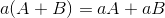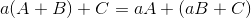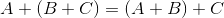Explanation:is the correct answer because it is the only answer that involves both the associative and distributive properties.

### Example Question #2 : Understanding The Multiplication Concept In Matrices As The Associative And Distributive Properties: Ccss.Math.Content.Hsn Vm.C.9

Which matrix when multiplied with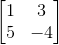will yield the same result regardless of the order in which they're multiplied?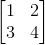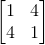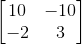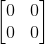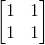Explanation:

The only matrix that works is, because regardless of the order of matrix multiplication, the result will always be.

### Example Question #1 : Understanding The Multiplication Concept In Matrices As The Associative And Distributive Properties: Ccss.Math.Content.Hsn Vm.C.9

Why doesn't the commutative property hold for matrix multiplication?

Commutative property doesn't work for regular multiplication

Order of multiplication matters

Order of multiplication matters

Explanation:

The reason that the commutative property doesn't apply to matrix multiplication is because order of multiplication matters. We multiply by the entry in the row of the first matrix by the entry in the column of the second matrix.

### Example Question #4 : Understanding The Multiplication Concept In Matrices As The Associative And Distributive Properties: Ccss.Math.Content.Hsn Vm.C.9

Which is an example of two matrices satisfying the distributive properties? Letbe a scalar, and,, andbe three unique matrices.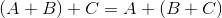Explanation:

The only answer that satisfies the distributive property is### Example Question #5 : Understanding The Multiplication Concept In Matrices As The Associative And Distributive Properties: Ccss.Math.Content.Hsn Vm.C.9

True or False: If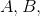andare square matrices, is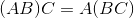?

False

True

True

Explanation:

Let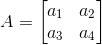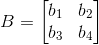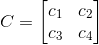.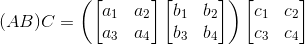Now do matrix multiplication inside the parenthesis.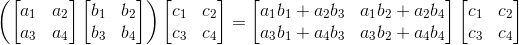Now multiply the result by the other matrix to get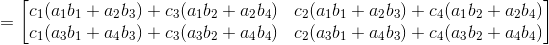Now lets do it from the other side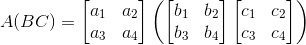Do the matrix multiplication inside the parenthesis first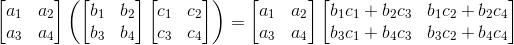Now multiply the result by the other matrix to get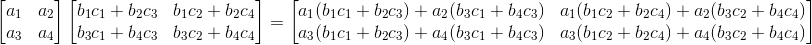If we rearrange the terms in this matrix we getSince these are the same matrix, we have evidence that the statement is true,.

### Example Question #1 : Understanding The Multiplication Concept In Matrices As The Associative And Distributive Properties: Ccss.Math.Content.Hsn Vm.C.9

True or False: The following matrix product is possible.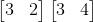True

False

False

Explanation:

The answer is false because the dimensions are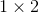for each matrix.

### Example Question #2 : Understanding The Multiplication Concept In Matrices As The Associative And Distributive Properties: Ccss.Math.Content.Hsn Vm.C.9

True or False:

The following matrix multiplication is possible.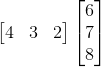True

False

True

Explanation:

The matrix multiplication is possible since the dimensions will work out. The result will be a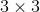since the dimensions are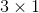, and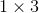.

### Example Question #3 : Understanding The Multiplication Concept In Matrices As The Associative And Distributive Properties: Ccss.Math.Content.Hsn Vm.C.9

True or False:

The following matrix multiplication is possible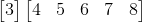False

True

True

Explanation:

The matrix multiplication is possible because the dimensions work out. The resulting matrix will be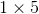, because the matrices are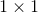, and.

### Example Question #4 : Understanding The Multiplication Concept In Matrices As The Associative And Distributive Properties: Ccss.Math.Content.Hsn Vm.C.9

True or False:

The following matrix multiplication is possible.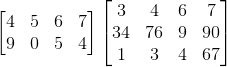True

False

The matrix multiplication is not possible because the dimensions do not work out. You can't multiply a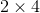and a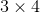matrix together.